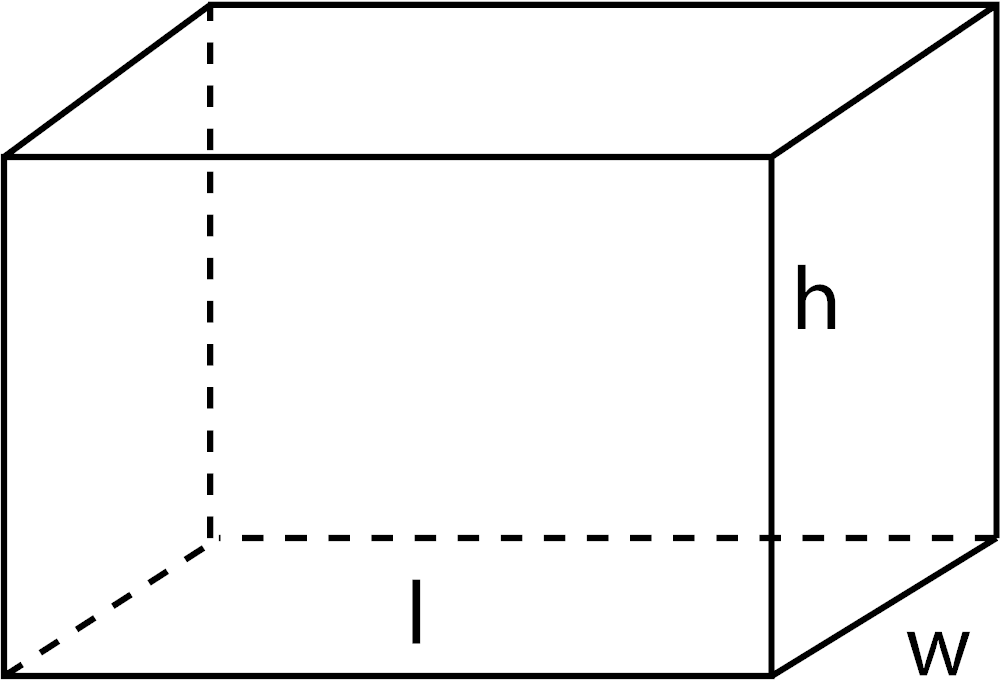# Length Converter

Convert a length measurement using the conversion calculator below.
Results:## Length Conversion Calculators

See a complete list of conversion calculators for each unit of length by selecting one below.
Most Popular
US Customary & Imperial Units
SI Units
Other Length Converters

## What is Length?

Length is defined as a measurement of distance between two points.

It can also be defined as the distance of the longest horizontal dimension of an object, from end to end. If the object is a rectangle, the length would be the distance of the longest edge of the rectangle.

Width is the distance of the shortest horizontal dimension of an object. Height is considered the distance of the vertical dimension of an object, and width is the distance of the shortest horizontal dimension of an object.## How to Convert Length Measurements

To convert measurements of length from one unit to another, convert the unit to a base unit using a known conversion formula, then convert from the base unit to the target unit of measurement. For length, meters are commonly used base unit since the meter is the SI unit of length.

So, to convert a unit of length, start by converting the measurement to meters first. Next, convert from meters to the target unit of measurement. You can use the conversion table below to find the conversion factor for the units you're converting.

For example, let's convert 18 inches to centimeters.

Step One: convert 18 inches to meters.

1 in = 0.0254 m
18 in = 18 × 0.0254 m
18 in = 18 × 0.4572 m

Step Two: convert 0.4572 meters to centimeters.

1 m = 100 cm
0.4572 m = 100 × 0.4572 m
0.4572 m = 45.75 cm

Thus, 18 inches is equal to 45.75 centimeters.

18 in = 45.75 cm

The length converter above along with our other unit conversion tools will help you easily convert a length measurement from one unit to another automatically. We have some similar measurement resources that you might also find interesting, such as our inch fraction calculator, printable tape measure, or our printable rulers.

## Different Measurements of Length

There are various measurement systems used to measure length; the most widely used today are the SI metric system and the US customary system.

### Units Used in the Metric System

The SI metric system is the most commonly used measurement system around the world, and it defines the meter (or metre) as the base unit of length. The SI metric system defines a list of prefixes to define other units of length in terms of the meter.

For instance, the centimeter uses the prefix "centi," which indicates that the unit is equal to 1/100 of a meter. The most commonly used units of length are the meter, kilometer, decimeter, centimeter, millimeter, micrometer, and nanometer.

### Units Used in the US Customary System

The US customary system is mostly used in the United States, but it's also used in a few other countries as well. US customary units were derived from the old British imperial units before the United States became an independent country.

Today, US customary units are defined in terms of the SI metric units of length, which was formalized in 1958 in a conference of English-speaking nations.

The units most commonly used in the US customary system are the inch, foot, yard, and mile, but other units such as the furlong, rod, chain, league, survey foot, and survey mile are used for surveying.

## How do you Measure Length?

To correctly measure length you'll need a measuring device of some kind. Rulers and tape measures are the most common measuring tools, but there are many ways to measure length, including without a tool.

Most tools offer a way to compare a scale on the tool to the real-world measurement to let you gauge the length. Some modern tools such as lasers, GPS, or lidar based imaging devices allow measuring without comparing a tool to a real-world measurement.

## Length Conversion Chart

The following table shows the conversion factors for the most common units of length.

Equivalent metric and imperial length measurements and conversion factors for common units
Convert From Mile Yard Foot Inch Kilometer Meter Centimeter Millimeter Micrometer Nanometer
1 mile = 1 mi 1,760 yd 5,280 ft 63,360 in 1.609344 km 1,609 m 160,934 cm 1,609,344 mm 1,609,344,000 µm 1,609,344,000,000 nm
1 yard = 0.000568 mi 1 yd 3 ft 36 in 0.000914 km 0.9144 m 91.44 cm 914.4 mm 914,400 µm 914,400,000 nm
1 foot = 0.000189 mi 0.333333 yd 1 ft 12 in 0.000305 km 0.3048 m 30.48 cm 304.8 mm 304,800 µm 304,800,000 nm
1 inch = 0.000015783 mi 0.027778 yd 0.083333 ft 1 in 0.0000254 km 0.0254 m 2.54 cm 25.4 mm 25,400 µm 25,400,000 nm
1 kilometer = 0.621371 mi 1,094 yd 3,281 ft 39,370 in 1 km 1,000 m 100,000 cm 1,000,000 mm 1,000,000,000 µm 1,000,000,000,000 nm
1 meter = 0.000621 mi 1.093613 yd 3.28084 ft 39.370079 in 0.001 km 1 m 100 cm 1,000 mm 1,000,000 µm 1,000,000,000 nm
1 centimeter = 0.0000062137 mi 0.010936 yd 0.032808 ft 0.393701 in 0.00001 km 0.01 m 1 cm 10 mm 10,000 µm 10,000,000 nm
1 millimeter = 0.00000062137 mi 0.001094 yd 0.003281 ft 0.03937 in 0.000001 km 0.001 m 0.1 cm 1 mm 1,000 µm 1,000,000 nm
1 micrometer = 0.00000000062137 mi 0.0000010936 yd 0.0000032808 ft 0.00003937 in 0.000000001 km 0.000001 m 0.0001 cm 0.001 mm 1 µm 1,000 nm
1 nanometer = 0.00000000000062137 mi 0.0000000010936 yd 0.0000000032808 ft 0.00000003937 in 0.000000000001 km 0.000000001 m 0.0000001 cm 0.000001 mm 0.001 µm 1 nm

Try our other measurement calculators.

## References

1. Weisstein, Eric W., Distance, MathWorld - A Wolfram Web Resource, https://mathworld.wolfram.com/Distance.html
2. Kenneth Butcher, Linda Crown, & Elizabeth J. Gentry, The International System of Units (SI) – Conversion Factors for General Use, National Institute of Standards and Technology, https://nvlpubs.nist.gov/nistpubs/Legacy/SP/nistspecialpublication1038.pdf
3. National Institute of Standards and Technology, SI Units – Length, https://www.nist.gov/pml/owm/si-units-length